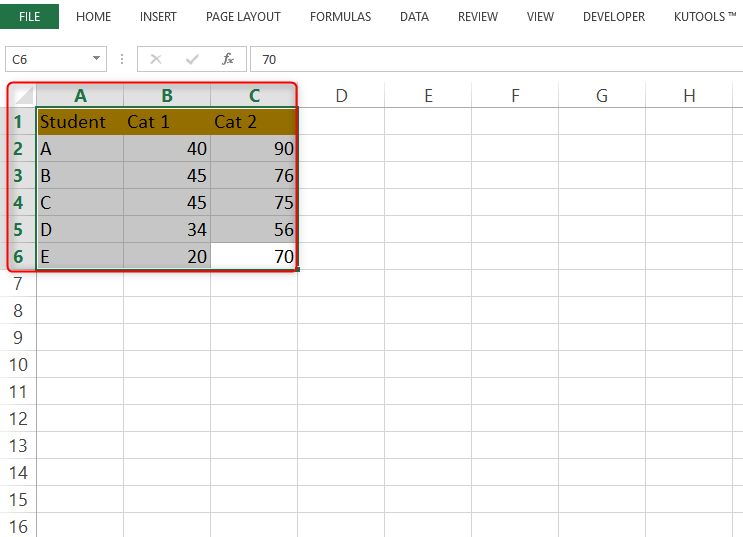# How to join two Excel Graphs together

Did you know you can join two excel graphs together? If you didn't know, now you know. However, many Excel users find this task to be challenging, which is not the case. Joining two graphs together not only saves the user time but also minimizes the space covered. Also, it may involve several workarounds which are simple to understand.

Let's discuss some of the common ways used to join two graphs in Excel.

## Using similar Chart series

Two graphs of different data can be drawn on the same graph using a similar chart type. However, the charts may have different colors for differentiating purposes.

Let us now discuss some of the steps to be followed when this method is used.

1. To get started, you should have the data of the two graphs ready.

In our example below, we have two data-column, cat 1&2, that represent graph 1 and graph 2 respectively.2. Once you've inputted the graph's data, highlight all the cells containing the graph's information.3. Then, locate and click on the "Insert" tab.

4. Then, from the Chart Section, choose the chart type you want. Each of the chart types has a drop-down menu that allows the user to access other types of charts within the selected chart type.In our example, let us choose the "2-D clustered column" chart. on clicking it, the highlighted graph is automatically converted to a graph.From the above, we can see each student has two vertical columns, each column representing each graph. Therefore, we have succeeded in combining two graphs into one.

## Using Copy and paste method

The copy and paste technique can also be used here to join two graphs into one. To do so, these steps are followed;

1. To get started, you should have the data of the two graphs ready.From the above, two columns should be represented by the graph. That is the cat 1 column and the cat 2 columns.

2. Once you've inputted the graph's data, highlight the first cell that contains the graph's information. In our case, we only highlight all the information apart from the column with Cat 2.3. Then, locate and click on the "Insert" tab.

4. Then, from the Chart Section, choose the chart type you want. Each of the chart types has a drop-down menu that allows the user to access other types of charts within the selected chart type.

After clicking, the graph of Cat 1 marks will be displayed.5. Unhighlight the previously selected data and highlight the second column.6. Then, Copy the data in column Cat 2 (Ctrl +c) and paste it (Ctrl + V) on the graph section. by doing so, the second graph will be displayed.## To change the chart type on the drawn graph

Once all your graph is drawn, you can go ahead and change the chart type of each graph. For example, you can make one a line graph whereas the second a bar graph.

Steps;

Right-click on the drawn graph and select the "Change series chart type."